Disclosure: This post may contain affiliate links, meaning when you click the links and make a purchase, we receive a commission.

# Excel Formula to Calculate Hours Worked Minus Lunch

In this article, I will show you the Excel formula to calculate hours worked minus lunch. The lunchtime can be in hours or it can be expressed as in starting and ending time. Based on different scenarios the formulas will be applied which will give you the number of hours worked excluding the lunch time.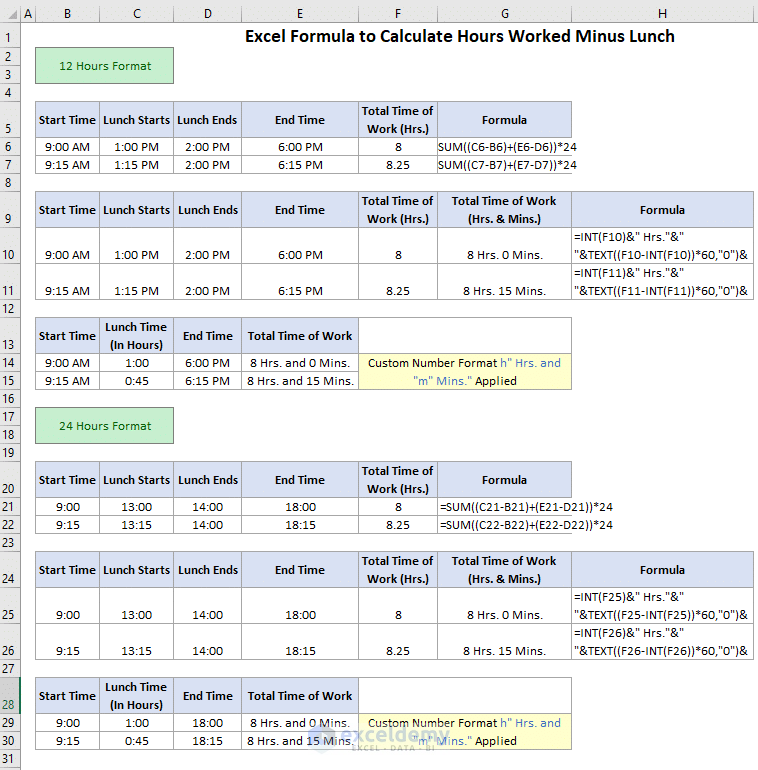## Calculate Hours of Work Where Only Hours will be Displayed

I will work here with 2 times format. In each case, the lunch starts and lunch ends time is given. You will get the hours results in fractions like 8.25, 7.75 etc. Don’t get confused if you find this fraction numbers. As we will find the differences at some different times, the fractional result is expected. The 8.25 means 8 hours 15 minutes where 7.75 means 7 hours and 45 minutes. In the same way, 8.50 means 8 hours 30 minutes. The formulas for calculating the number of working hours are placed for two different time formats. You will get the same results from both the formats.

### 12 Hour Time Format

In 12 hours’ time format, the AM/PM time formatting is applied without the Total Time of Works (Hrs.) column. The formula which is applied here is =SUM((D3-C3)+(F3-E3))*24. You can also apply the formula =SUM((F3-C3)-(E3-D3))*24 which will give you the same result.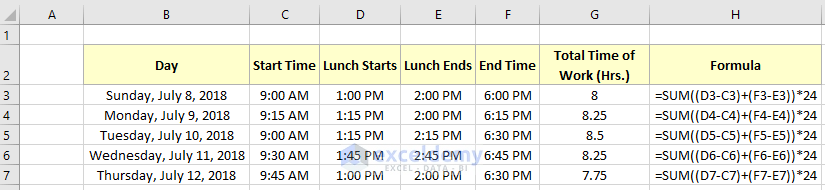### 24 Hour Time Format

In 24 hours’ time format, the 13: 30-time formatting is applied without the Total Time of Works (Hrs.) column. The formula which is applied here is =SUM((D3-C3)+(F3-E3))*24. You can also apply the formula =SUM((F3-C3)-(E3-D3))*24 which will give you the same result.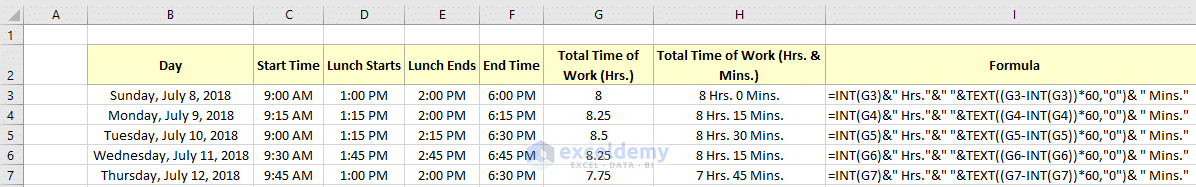## Calculate Hours of Work Where Hours and Minutes will be Displayed

To avoid the fraction numbers, you can display your results as Hours and Minutes together. For this, you need an extra formula. Before that, use the previous formulas to calculate the total hours of work in hours. Here the formatting of 12 hours and 24 hours are formatted as before. I am showing you the 12 hours format style. The formula is the same for both.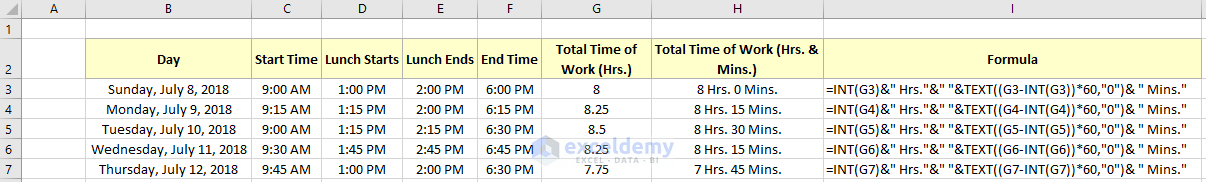In the above picture, the hours of work calculated using the formula, =SUM((D3-C3)+(F3-E3))*24 in the Total Time of Work (Hrs.) column. To display it as hours and minutes the formula, =INT(G3)&” Hrs.”&” “&TEXT((G3-INT(G3))*60,”0″)& ” Mins.” was used.

## Display Hours and Minutes of Work Where Lunch Hour is Given

Instead of Lunch Starts and Lunch Ends time, only the Lunch Time (In Hours) can be given. In this case, showing the hours of work becomes difficult due to the formatting. To avoid this problem follow the below procedure.

• In cell F3, write down the formula =(E3-C3)-D3.
• Now, double-click on the fill handle to copy this formula for the rest of the cells of the column.
• After this, select the cells where this formula is applied and press on to the right button of your mouse to select the Format Cells option.
• In the Format Cells dialogue box, select Custom under the Category section and write down `h" Hrs. and "m" Mins." `In the Type section.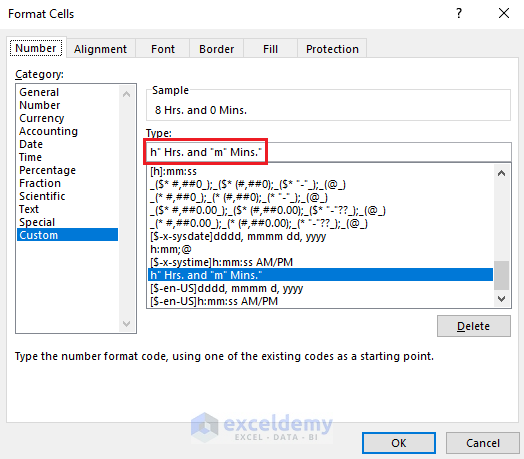• Now after pressing OK, you will get to see the below result.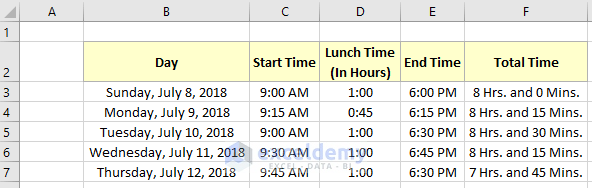## Conclusion

By reading this article you will certainly get an idea on how we can use the Excel formula to calculate hours worked minus lunch. This is a very easy task but with different time formats sometimes it becomes difficult. I hope after reading this article you will not face any difficulties while calculating the difference several times.

## Related Articles

Hello!

Welcome to my Profile. Here I will be posting articles related to Microsoft Excel. I have completed my BSc in Electrical and Electronic Engineering from American International University-Bangladesh. I am a diligent, goal-oriented engineer with an immense thirst for knowledge and attitude to grow continuously. Continuous improvement and life-long learning is my motto.

1. ReplyNollie Jul 30, 2018 at 4:13 PM

People normally pay me for this and you are giving it away!

2. ReplyJim Nov 7, 2018 at 9:56 PM

This is a lot of detail, well done.

Only problem is, you’re not showing the formula for the F column – Excel doesn’t handle sum/subtraction for time correctly on its’ own.

• ReplyDec 25, 2018 at 3:40 PM

Thanks.

3. ReplyBill Feb 13, 2019 at 8:16 PM

I have taken several of your courses and really appreciate these frequent tips. Excel is so powerful and extensive that I doubt I will ever use it to its potential but I do appreciat these insightful learning aids. Thank you so very much!

• ReplyFeb 14, 2019 at 4:26 PM

You’re most welcome 🙂 I am glad to know that our tutorials add some value to your life.

4. ReplyWayne Williams Jun 26, 2019 at 1:45 PM

Hi there this is the most easy to follow advice out there so thankyou so much, please can I ask when you have a start time, total lunch time and end time and then the total hours and minutes worked such as =(E3-C3)-D3, how to I convert this into a fraction> You do have have this as a end formual above where you have start time, start lunch, end lunch and end time and the end formual is worked out in hours like 6.75hrs etc, but I need it when I only have start and finidhs time and, lunch break total – any advice most welcome 🙂

• ReplyLarry A Joyner Dec 1, 2019 at 8:40 PM

=((E3-C3)-D3)*24